Determination of Angular Velocity

Pritha Mandal, Laura Pennington, Matthew Bergstresser
• Author
Pritha Mandal

Pritha is an academic editor and technical writer. She holds a Master's degree in Physics, with specialization in Astrophysics. Pritha has edited hundreds of physical science research papers and has co-authored a paper on radio astronomy.

• Instructor
Laura Pennington

Laura received her Master's degree in Pure Mathematics from Michigan State University, and her Bachelor's degree in Mathematics from Grand Valley State University. She has 20 years of experience teaching collegiate mathematics at various institutions.

• Expert Contributor
Matthew Bergstresser

Matthew has a Master of Arts degree in Physics Education. He has taught high school chemistry and physics for 14 years.

What is angular velocity? Find out with an angular velocity definition and examples. Learn how to find angular velocity using the angular velocity formula. Updated: 07/27/2021

Show

What is Angular Velocity?

To understand the concept of angular velocity, it is important to first know what rotation is. Think of a spinning top or a windmill. These objects are rigid bodies and rotate about a fixed axis. A rigid body is one that rotates with all of it locked together, without any change in the shape of the body, and a fixed axis is an axis that does not move. In the case of the spinning top, the axis of rotation passes through the body of the top itself; for the windmill, the blades rotate around an axis that passes through the rotor. In both cases, the objects are exhibiting rotational motion. Some other examples of bodies exhibiting rotational motion are:

• A spinning ice skater
• The rotation of the earth
• A rotating ceiling fanThe velocity associated with rigid bodies as they exhibit rotation about a fixed axis is called angular velocity. Angular velocity is generally represented by the Greek letter omega ({eq}\mathbf{\omega} {/eq}).

Angular Velocity vs Linear Velocity

Angular velocity in rotational motion is analogous to linear velocity in translational motion. When a body travels in a straight line, the change in its linear position per unit time gives the linear speed; when the direction of motion is also considered, the linear velocity of the body is defined. Similarly, when a body exhibits rotational motion, the change in its angular position per unit time along with the direction of motion gives the angular velocity of the body.

The angular velocity and linear velocity of a body can be related to each other. If a body is rotating about a fixed axis with an angular velocity of {eq}\omega {/eq}, the linear speed {eq}v {/eq} of any point within the body at a distance {eq}r {/eq} from the axis of rotation is given by

{eq}v=\omega r {/eq}.An error occurred trying to load this video.

Try refreshing the page, or contact customer support.

Coming up next: Average Velocity: Definition, Formula & Examples

You're on a roll. Keep up the good work!

Replay
Your next lesson will play in 10 seconds
• 0:00 Linear Velocity
• 2:22 Angular Velocity
• 3:14 Angular Velocity Formulas
• 6:11 Lesson Summary
Save Save

Want to watch this again later?

Timeline
Autoplay
Autoplay
Speed Speed

Angular Velocity Formula

Before we look at the formula for angular velocity, let's first understand the more fundamental concepts of angular position and angular displacement. Consider the figure below.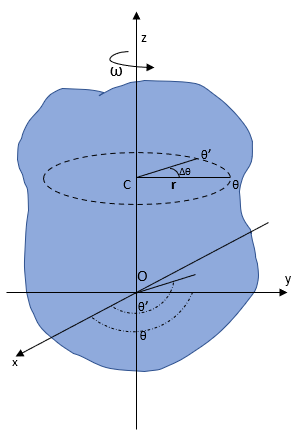A rigid body is rotating about the z-axis with angular velocity {eq}\omega {/eq}. Every particle of the rigid body rotates about the z-axis and traces a circle lying on a plane perpendicular to the axis of rotation (z-axis) with its center on the axis. Now, consider a rigid body particle at a distance {eq}r {/eq} from the axis. Its angular position with respect to a reference line (x-axis) is {eq}\theta {/eq}. As time passes, the particle rotates about the z-axis and after time {eq}\Delta t {/eq}, its angular position becomes {eq}\theta' {/eq}. Thus, the angular displacement of the particle, {eq}\Delta \theta =\theta '-\theta {/eq}.

The angular velocity is defined as the angular displacement per unit time. Therefore, the angular velocity of the particle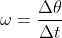The angular position (and angular displacement) is measured in units of radians (rad). A radian is a measure of an angle. It is defined as the ratio of an arc of a circle to the radius of the circle. Thus, 1 rad is the angle for which the length of the arc is equal to the radius of the circle.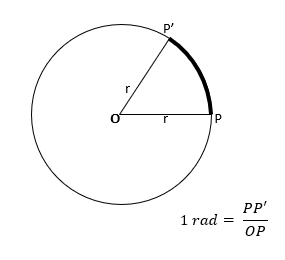Another common measure of angles is degrees ({eq}^{\circ} {/eq}). The conversion of the unit of angles from radians to degrees and from degrees to radians is pretty simple. If we go around an entire circle, the length of the arc traversed is equal to the circumference of the circle, i.e., {eq}2\pi r {/eq}. So, the number of radians in the whole circle is,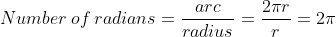Now, we know that the total angle of a circle in degrees is {eq}360^{\circ} {/eq}.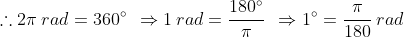The table below shows some measures of angles in radians and degrees.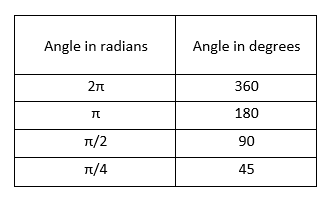Angular Velocity Units

Angular velocity is the angular displacement of a body per unit of time. As radians (rad) and seconds (s) are the SI units of angular displacement and time, respectively, the SI unit of angular velocity is radian per second (rad/s). Angular velocity is also sometimes expressed in terms of revolution per second (rev/s). Another unit of angular velocity is one that was commonly used when vinyl records were in, the unit of revolution per minute (rev/min), commonly termed rpm.

We know, {eq}1\: rev=2\pi \: rad {/eq}. Thus, {eq}1\: rev/s=2\pi \: rad/s {/eq}.

Again, {eq}1\:s=\frac{1}{60}\:min {/eq}. Thus, {eq}1\: rpm=\frac{2\pi}{60} \: rad/s {/eq}.

How to Find Angular Velocity

Now that we've learned the formula and units of angular velocity, let's look at a few interesting examples.

Example 1

Consider a Ferris wheel at a carnival. If the Ferris wheel completes one rotation in {eq}3\:min {/eq}, what is its angular velocity in rad/s and rpm?

We have,Here, {eq}\Delta \theta=2\pi {/eq} (one full rotation) and {eq}\Delta t=3\: min=\left ( 3\times 60 \right )\: s=180\: s {/eq}.

Thus, the angular velocity of the Ferris wheel in rad/s is,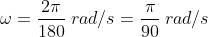Now,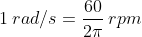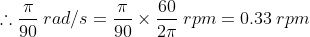Example 2

A mountain bike has wheels of diameter {eq}61\:cm {/eq}. An amateur biker rides for {eq}1.22\:m {/eq} to test out the bike and finds that it takes him {eq}2\:s {/eq}. What is the angular velocity of the wheel of the bike?

We have, angular velocity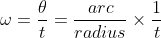Here, {eq}arc=1.22\:m=122\:cm {/eq}, {eq}radius=61/2\:cm {/eq}, and {eq}t=2\:s {/eq}.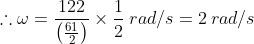Example 3

What should the minimum angular velocity of a race car be so that it has a speed of {eq}150\:mph {/eq} or above when racing along a circular track of radius {eq}0.3\: miles {/eq}?

We have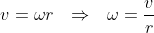Here {eq}v=150\:mph {/eq} and {eq}r=0.3\: miles {/eq}.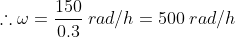Thus, the race car should have a minimum angular velocity of {eq}500\:rad/h {/eq}.

How Angular Velocity is Used

You might remember how at the beginning of the lesson we said that angular velocity involves both magnitude and direction. In the above examples, the angular velocity we determined was actually the magnitude of angular velocity. The direction of the angular velocity vector can be determined by the right-hand rule.

Right-hand rule

According to the right-hand rule, if you curl your right hand around the axis of rotation of a rotating body with your fingers pointing in the direction of rotation, the direction of your extended thumb in the direction of the angular velocity vector. Thus, here, the rigid body rotates around the direction of the angular velocity vector.

To unlock this lesson you must be a Study.com Member.

Angular Velocity

Angular velocity describes the rate at which an object rotates and the direction it rotates. It is standard convention for the counterclockwise direction to be the positive direction. Let's practice some angular velocity problems. For constant angular velocity the ratio of the angular displacement (θ) to the time of motion is the angular velocity.

Practice problems

1. A ball on a string is rotating clockwise and it covers 20 radians in 5 seconds. What is its angular velocity?

2. A merry-go-round is rotating counterclockwise. It makes 10 revolutions in 23 seconds. What is its angular velocity?

3. How many revolutions does a turntable rotate through if it is moving at 32 rad/s and has been turning for 4 minutes?

Solutions

1.

• t = 5 sec
• ω = ?

ω = θ / t

ω = 20 rad / 5 s

2.

• θ = 10 rev x (2π rad) / (1 rev) = 62.83 rad
• t = 5 s
• ω = ?

ω = θ / t

ω = 62.83 rad / 5 s

3.

• θ = ?
• t = 4 min x (60 seconds) / (1 min) = 240 s

ω = θ / t

32 rad/s = θ / 240 s

Register to view this lesson

Are you a student or a teacher?